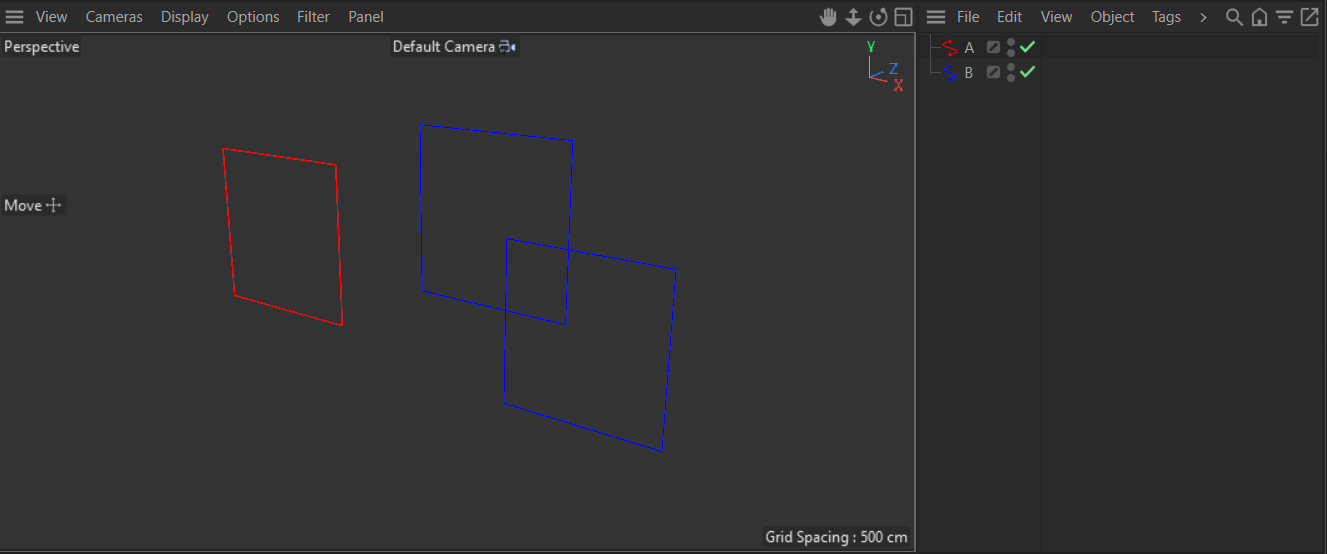# GetSegmentCount() returning 0 for a spline object with only one segment

Hi,

I am having a problem with GetSegmentCount() returning 0 for a spline object with only one segment, such as a Rectangle Spline. Incidentally, the correct value is returned for Text Spline objects.

The test script is here:

``````import c4d

def main():
spline = op.GetRealSpline()
scnt = spline.GetSegmentCount()
print(scnt)

if __name__ == '__main__':
main()
``````

When I select Rectangle spline object and run the script up, it returns 0 which is not correct.
If I am wrong, please let me know. Thank you!

Hello @kng_ito,

thank you for reaching out to us. The solution to your problem is that you should get the cache of `op` instead of the real spline. But this seems to be some kind of regression. The underlying reason is that `GetSegmentCount()` does nothing else than counting the number of data blocks for the first `Tsegment` tag present on the `Spline/LineObject` it is being called on. The problem is: That tag is not present for splines with a segment count less than two.

For this scene,and this script:

``````import c4d

op: c4d.BaseObject

def main():
"""
"""
if op is None:
return

print (f"\nData for {op.GetName()}:\n")

for label, node in [(f"{op.GetName()}", op),
(f"{op.GetName()}(Cache)", op.GetCache())]:
if node is None:
continue

print (f"\t{label}.GetSegmentCount() = {node.GetSegmentCount()}")

tags: list[c4d.BaseTag] = node.GetTags()
print (f"\t{label}.GetTags() = [")
for tag in tags:
print (f"\t\t{tag}")
print ("\t\t]\n")

if __name__ == '__main__':
main()
``````

running the script for both the spline objects A (red, one segment) and B (blue, two segements) will spit out the following output (minus the comments :) ) :

``````
Data for A:

A.GetSegmentCount() = 0
A.GetTags() = [
# This is the problem here, there is no Tsgement tag on the spline and GetSegmentCount(),
# its underlying generic implementation, will therefore return `0` as it cannot find that tag.
<c4d.PointTag object called  with ID 5600 at 1823374312960>
]

A(Cache).GetSegmentCount() = 1
A(Cache).GetTags() = [
<c4d.VariableTag object called  with ID 5712 at 1823374308864>
<c4d.VariableTag object called  with ID 5680 at 1823374308992>
<c4d.SegmentTag object called  with ID 5672 at 1823374306048>
<c4d.PointTag object called  with ID 5600 at 1823374305856>
]

Data for B:

B.GetSegmentCount() = 2
B.GetTags() = [
<c4d.SegmentTag object called  with ID 5672 at 1823374290496>
<c4d.PointTag object called  with ID 5600 at 1823374290368>
]

B(Cache).GetSegmentCount() = 2
B(Cache).GetTags() = [
<c4d.VariableTag object called  with ID 5712 at 1823374286016>
<c4d.VariableTag object called  with ID 5680 at 1823374283264>
<c4d.SegmentTag object called  with ID 5672 at 1823374283072>
<c4d.PointTag object called  with ID 5600 at 1823374281216>
]
``````

So, for now, you should call `GetSegmentCount()` on the `LineObject` in the cache of the `SplineObject`. But as I said, I personally would consider this a regression/bug. I will talk with the developers, but I faintly remember that with `Tsgement` some oddities were intended because of the new modelling kernel. So we might consider this behavior intended. I will keep this thread updated.

edit: So, I briefly talked with one of the devs, and they somewhat consider this intended, so this will likely not be changed in the close future.

Cheers,
Ferdinand

MAXON SDK Specialist
developers.maxon.net

Hi @ferdinand ,

Thanks to your answer, I was able to work around the problem with GetSegmentCount() by using GetCache(), but it seems that GetSegment() does not work correctly as well when the number of segments is less than 2.

Result of running against a single segment spline:

``````>>> op.GetCache().GetSegment(id=0)
AttributeError: 'c4d.LineObject' object has no attribute 'GetSegment'

>>> op.GetRealSpline().GetSegment(id=0)
IndexError: segment index out of range
``````

However, I know that it is not a big problem because the information returned by GetSegment() is basically just the number of vertices belonging to that segment, so I can avoid the problem by doing the following.

``````if segment_count > 1:
segment = spline.GetSegment()
else:
# If there is only one segment, it equals the number of vertices in the entire spline.
segment = {"cnt": spline.GetPointCount(), "closed": spline.IsClosed()}
``````

Even if this behavior is also intended, I personally felt it was unkind, so I am reporting it.
At least the problem is solved, thanks anyway.

Hello @kng_ito,

I agree that it is odd, or as you nicely put it, unkind. The problem is also that the meaning of segments in the context of a `SplineObject` (the data count of the `Tsegment` tag) and a `LineObject` (the data count of its `Tline` tag) is being mixed up.

`````` B(Cache).GetSegmentCount() = 2                            <- A LineObject instance.
B(Cache).GetTags() = [
<c4d.VariableTag object called  with ID 5712 ...   <- The Tline tag.
<c4d.VariableTag object called  with ID 5680 ...
<c4d.SegmentTag object called  with ID 5672 ...    <- The Tsegment tag for the host.
<c4d.PointTag object called  with ID 5600 ...
]
``````

The problem of this design is that `LineObject.GetSegmentCount` returns the segment count in the sense of its host object, the `SplineObject`, as the number of disjunct curve sections in the spline (segment tag). The segments in the sense of a `LineObject`, the number of line segments the hosting spline object has been quantized into in the `LineObject` (line tag), is only indirectly accessible over getting the `Tline` tag of that object. Which in turn might confuse users why they cannot get a specific segment as you wanted to.

But this design was apparently intentional and aims at minimizing the number of tags in the traverseable scene-graph (things inside caches are out of sight so to speak and not a problem). It is therefore unlikely that we will change this. The best I can do for now, is adding a note to `LineObject.GetSegmentCount` and `SplineObject.GetSegmentCount` which hints at the problem.

Cheers,
Ferdinand

MAXON SDK Specialist
developers.maxon.net